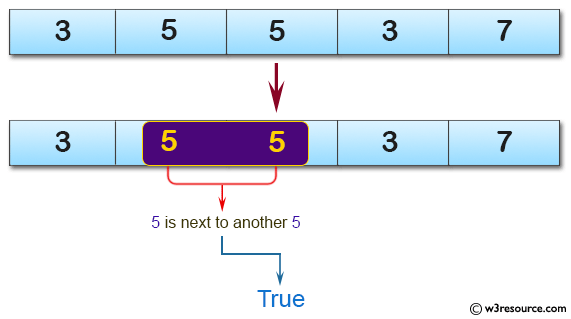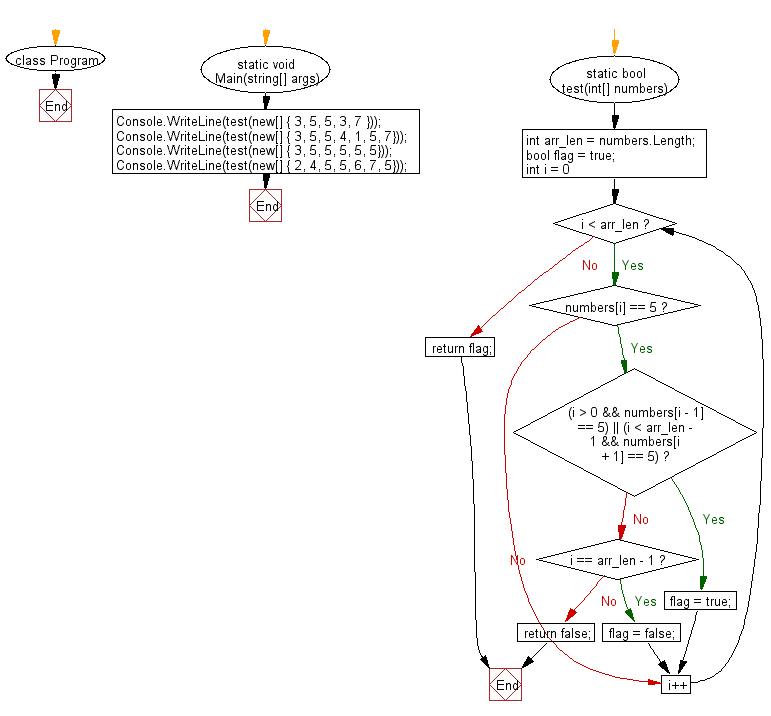﻿ C# - Check in an array if every 5 appears next to another 5# C# Sharp Basic Algorithm Exercises: Check a given array of integers and return true if every 5 that appears in the given array is next to another 5

## C# Sharp Basic Algorithm: Exercise-124 with Solution

Write a C# Sharp program to check a given array of integers and return true if every 5 that appears in the given array is next to another 5.

Pictorial Presentation:Sample Solution:

C# Sharp Code:

``````using System;
namespace exercises
{
class Program
{
static void Main(string[] args)
{
Console.WriteLine(test(new[] { 3, 5, 5, 3, 7 }));
Console.WriteLine(test(new[] { 3, 5, 5, 4, 1, 5, 7}));
Console.WriteLine(test(new[] { 3, 5, 5, 5, 5, 5}));
Console.WriteLine(test(new[] { 2, 4, 5, 5, 6, 7, 5}));
}
static bool test(int[] numbers)
{
int arr_len = numbers.Length;
bool flag = true;

for (int i = 0; i < arr_len; i++)
{
if (numbers[i] == 5)
{
if ((i > 0 && numbers[i - 1] == 5) || (i < arr_len - 1 && numbers[i + 1] == 5)) flag = true;
else if (i == arr_len - 1) flag = false;
else return false;
}
}
return flag;
}
}
}
```
```

Sample Output:

```True
False
True
False
```

Flowchart:C# Sharp Code Editor:

Improve this sample solution and post your code through Disqus

What is the difficulty level of this exercise?

Test your Programming skills with w3resource's quiz.

﻿